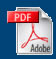Home English Maths Science ICT History Geography Art Year Six Revision Maths APP
Home > Maths APP >Level 2
 Level 2 Level 3 Level 4 Level 5 Level 6 Level 7 Level 8

Assessing Pupil Progress at Level 2
Criteria Introduction Practice Assessment Back-up Action
Select the mathematics they use in some activities (Problem Solving)Discuss their work using mathematical language (Communicating)
Begin to represent their work with simple symbols and diagrams (Communicating)
Explain why an answer is correct (Reasoning)
Predict what comes next in a simple number, shape or spatial pattern sequence and give reasons for their opinions (Reasoning)
Count sets of objects reliably (Numbers and the Number System)
Recognise sequences of numbers including odd and even numbers (Numbers and the Number System)
Begin to use halves and quarters (Fractions)Relate the concept of half of a small quantity to the concept of half of a shape (Fractions)Use the knowledge that subtraction is the inverse of addition (Operations and the relationships between them)Understand halving as a way of "undoing" doubling and vice versa (Operations and the relationships between them)Use mental recall of addition and subtraction facts to ten (Mental Methods)Use mental strategies to solve number problems including those involving money and measures (Mental Methods)Choose appropriate operation when solving addition and subtraction problems (Solving Numerical Problems)
Solve number problems involving money and measures (Solving Numerical Problems)
Record their work in writing (Written Methods)Use mathematical names for common 3D and 2D shapes (Properties of Shape)
Describe their properties including numbers of sides and corners (Properties of Shape)Describe the position of objects (Properties of Position and Movement)Distinguish between straight and turning movements (Properties of Position and Movement)
Recognise right angles in turns (Properties of Position and Movement)
Understand angle as a measurement of turn (Measures)
Begin to use everyday non-standard and standard units to measure length and mass (Measures)Begin to use a wider range of measures (Measures)Sort objects and classify them using more than one criteria (Processing and Representing Data)Understand vocabulary related to handling data (Processing and Representing Data)Collect and sort data to test a simple hypothesis (Processing and Representing Data)Record results in simple lists, tables, pictograms and block graphs (Processing and Representing Data)Communicate their findings using simple lists, tables, pictograms and block graphs that they have recorded (Interpreting Data)Securing Level Two

Please ensure you use your judgement in terms of assessing pupil progress. Webpage is developed as a tool to help you to do that, but no responsibility will be accepted in relation to incorrect judgements made as a result of using this web page. The use of this webpage is provided free of charge and in good faith. However, the developer is no expert.